Previous: 3.2.1 Distribution Families Up: 3.2 Maximum Entropy Closure Next: 3.2.3 Diffusion Closure

## 3.2.2 Critique and Modifications

The maximum entropy method has been criticized by Junk [JU00], see also [BBB00] and [Tag03]. For the semiconductor Boltzmann equation with parabolic bands the equilibrium states are located on the boundary of the domain of definition of the maximum entropy system. For the maximum entropy closure involving moments of fourth order or higher, the flux function of the maximum entropy system is singular in equilibrium points. Arbitrarily close to equilibrium, the maximal characteristic velocity becomes arbitrarily large. These results also imply, that the maximum entropy closure does not always exist.

The maximum entropy closure is not invariant under a nonlinear transformation of the random variables. For example, maximizing the entropy for the distribution of the random variable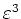corresponding to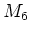gives a different closure. To apply the maximum entropy closure a set of natural'' random variables has to be distinguished.

Furthermore, in the maximum entropy closure as applied in [ARR00] it is assumed that, e.g., the first five moments are known and then the sixth moment is estimated. No knowledge is assumed about the higher order moments. However, this ignores available information about the sixth moment - as this moment enters into the equation for the fourth moment.

To include this available information we propose the following modified maximum entropy closure: We apply the maximum entropy principle to the class of all solutions of the under-determined equation system (4 unknowns: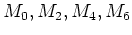, 3 equations for: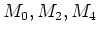). The entropy which is to be maximized is the global entropy of the six-dimensional function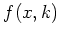. In this wayis not pre-eliminated and is treated on a same footing with the lower even moments. However, carrying through this approach leads to an even more complicated system of equations.

This is also the natural way in which the infinite hierarchy of moment equations (as discussed in Section 2.3.5) should be closed, i.e., of all solutions of the infinite hierarchy choose the one with maximum entropy.